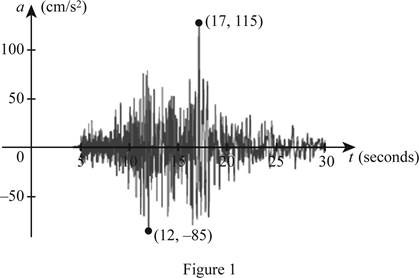# To estimate: The range of the vertical ground acceleration functions at USC during the Northridge earthquake.### Single Variable Calculus: Concepts...

4th Edition
James Stewart
Publisher: Cengage Learning
ISBN: 9781337687805### Single Variable Calculus: Concepts...

4th Edition
James Stewart
Publisher: Cengage Learning
ISBN: 9781337687805

#### Solutions

Chapter 1.1, Problem 3E
To determine

## To estimate: The range of the vertical ground acceleration functions at USC during the Northridge earthquake.

Expert Solution

The range of the vertical ground acceleration function is 85a(t)115 .

### Explanation of Solution

Given:

The graph which was recorded by an instrument operated by the California Department of Mines and Geology at the University Hospital of the University of Southern California in Los Angeles is shown below in Figure 1.Estimation:

In Figure 1, the x-axis represents time in seconds and the y-axis represents the vertical ground acceleration during the Northridge earthquake in cm/s2.

It is observed from Figure 1 that the minimum point occurs at (12, −85) and the maximum point occurs at (17, 115).

Since, the range is the set of all y-values of the graph, the range of the vertical ground acceleration function is estimated to 85a(t)115 . Thus, the range of the vertical ground acceleration is [−85, 115].

### Have a homework question?

Subscribe to bartleby learn! Ask subject matter experts 30 homework questions each month. Plus, you’ll have access to millions of step-by-step textbook answers!